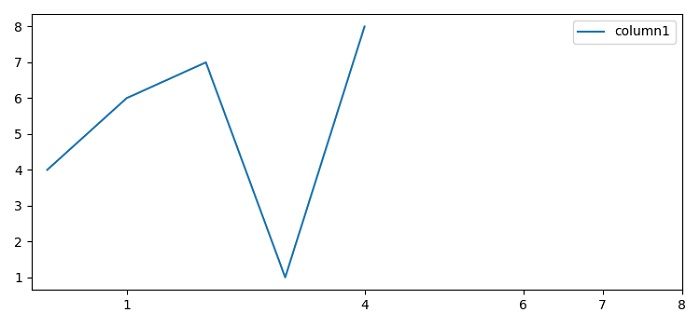# How to set Dataframe Column value as X-axis labels in Python Pandas?

To set Dataframe column value as X-axis labels in Python Pandas, we can use xticks in the argument of plot() method.

## Steps

• Set the figure size and adjust the padding between and around the subplots.

• Make a dataframe using Pandas with column1 key.

• Plot the Pandas dataframe using plot() method with column1 as the X-axis column.

• To display the figure, use show() method.

## Example

import pandas as pd
from matplotlib import pyplot as plt
plt.rcParams["figure.figsize"] = [7.50, 3.50]
plt.rcParams["figure.autolayout"] = True
data = pd.DataFrame({"column1": [4, 6, 7, 1, 8]})
data.plot(xticks=data.column1)
plt.show()

## OutputUpdated on: 01-Jun-2021

6K+ Views